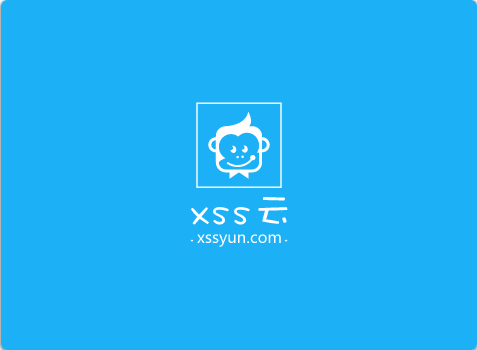# 计算广告丨《互联网广告算法和系统实践》读书笔记站长

AVR单片机教程——UART进阶

## 第一部分 互联网广告简介

### 1.1 广告简介

• 广告是由已确定的出资人通过各种媒介进行的有关产品（商品、服务和观点）的、有偿的、有组织的、综合的、劝服性的非人员的信息传播活动。

• 传统广告业务包括三方角色：广告主、媒体、普通受众
• 广告历史

### 1.2 互联网广告

• 显示广告、合约广告、定向广告、受众定向技术
• 担保式投放
• 竞价广告、广义二阶拍卖GSP、广义一阶拍卖GSP
• 搜索广告、上下文广告、实时竞价
• 广告交易平台、需求方平台、提供方平台
• 计费方式：点击付费CPC、销售付费CPS、千次展现付费CPM

• 条幅广告
• 邮件直接营销广告
• 富媒体广告
• 视频广告
• 文字链广告
• 社交广告
• 移动端广告

### 1.4 有效性模型• 曝光：位置很重要。
• 关注：广告创意吸引人，借助算法定向精准投放
• 理解：让用户迅速理解广告
• 信息接受：研究人群行为，还要借助心理学
• 保持：追求中长期转化
• 购买：最终决策，给用户提供更多更好的选择

### 1.5 计费模式

• CPM：Cost Per Mile，按照千次展现计费，展现之后的效果广告平台不保证。适合品牌广告。
• CPT：Cost Per Time，按照单位时间计费。为了增加曝光度。
• CPC：Cost Per Click，按照千次点击计费，广告系统要负责对点击率CTR预估；广告主可以参与竞价。关注点击率，但不关注广告实际成交情况，风险由广告主承担。
• CPS：Cost Per Sale，关注转化率，对广告主有利。

### 1.6 机制设计

#### 1.6.1 广告位拍卖

• n个广告A，m个广告位S，m＜n，广告位拍卖从A中选取m条广告，依次放置到S中。
• 拍卖竞争除了展现机会，还包括S中排序位置。
• 广告位拍卖过程分为广告排序和广告扣费。广告排序考虑展现哪些广告，广告扣费考虑收取多少费用。
• 广告拍卖是多次重复博弈，纳什均衡点、本地无嫉妒均衡、占优策略、激烈相容

#### 1.6.2 广义一阶价格GFP

• 广告展现不扣费
• 一阶价格是指广告主本身出价。
• 不存在纳什均衡点，竞价机器人导致广告系统收益大大降低。

#### 1.6.3 广义二阶价格GSP

• 对应第i位广告，若发生点击，GSP收取第i位广告主，第i+1位广告竞价加上一个货币最小值。
• 若广告主降低竞价，其展现位置不变时，不会降低收费。下家没有降低竞价行为，导致上家付出更多点击费用。存在本地无嫉妒均衡。
• 均衡报价：n个广告A，每条广告价值V，m个广告位S，每个位置点击率X，每个位置当前竞价P。对于任意广告位i上获得位置的广告j有$$(v_j-p_i)x_i\ge(v_j-p_{i-1})x_{i-1}$$
• 均衡点不唯一，不是激励相容。
• 现实中广告扣费要考虑点击率因素。对于广告i，广告系统预估i在当前场景点击率ai，结合竞价bi，计算每千次展现期望收益$$ecpm_i=a_ib_i$$，随后将广告集合按照$$ecpm$$排序，排序靠前若干条用于展现。计费时，若第i个广告被点击，从广告主扣除费用为$$\frac{a_{i+1}b_{i+1}}{a_i}+最小货币单位$$
• 若广告点击率越高，广告主扣除费用越少，激励用户优化广告质量提高广告点击率。

#### 1.6.4 VCG机制

• 目标为最大化社会价值
• 有n个广告A，广告竞价为V，广告平台提供S广告位，每个位置点击率X，每条广告真实价格V。当广告i不参与关键字拍卖，排在i后的广告主i+1,…,m可获得的预期总收益为

$x_iv_{i+1}+x_{i+1}v_{i+2}+…+x_mv_{m+1}$

• 由于$$a_i$$参与，广告获得价值为

$x_{i+1}v_{i+1}+x_{i+2}v_{i+2}+…+x_mv_{m}+x_{m+1}v_{m+1}$

• 通常只有m个广告位，所以$$x_{m+1}=0$$，如果$$a_i$$被点击，那么它要付出代价是给社会其他个体带来的利益损失

$(x_i-x_{i+1})v_{i+1}+(x_{i+1}-x{i+2})v_{i+2}+…+(x_{m-1}-x_m)v_m+(x_m-x_{m+1})v_{m+1}$

• VCG相比GSP优势
1. VCG激烈相容，按照真实估价出价是最优选择
2. VCG机制最大化社会价值，有利于广告主
3. VCG存在纳什均衡，均衡点唯一
• 缺点
1. 计算困难，可解释性差
2. 相同竞价情况VCG扣费低于GSP机制，广告系统不愿意降低收入

### 1.7 技术课题

• 优化目标
• 计算广告学：找到用户、上下文和合适广告间最佳匹配。
• 互联网广告算法核心问题：根据用户、环境、广告全部有效消息，找到最合适的投放策略和模型，兼顾浏览者、广告主、广告平台的最大利益，并不断调整。
• 用户U、环境C、广告A，算法目标函数为$$F(U,C,A)$$
• 对于广告主，关注投资回报率ROI，$$F=\frac{\sum_iReturn_i}{\sum_iInvest_i}$$
• 对于用户，$$F=\frac{\sum_iClick_i}{\sum_iImpression_i}$$
• 对于广告系统，$$F=CTR*PPC*Discount$$$$CTR$$是点击率，$$PPC$$是点击收费，$$Discount$$业务因子。
• 搜索引擎技术
• 广告系统和搜索引擎相似，广告系统没有爬虫模块，通过规范化步骤获取。
• 存储技术和实时计算技术
• 需要一个适合大规模读写的存储系统，需要实时计算系统。
• 推荐技术
• 点击率预估CTR Prediction
• 广告主工具
• 对广告主，广告系统提供推广工具和统计工具。
• 系统架构简介
• 前端引擎：接受网页发来广告请求，初步处理发给后端，拿到结果拼接返回请求者，这是在线部分Online
• 检索引擎：关注效率和性能，Online
• 实时点击率预估服务：对广告打分，一维、二维分数，Online
• 广告主操作消息更新服务：广告主有权随时更改广告竞价，Online
• 用户行为数据收集和更新系统：一般是Online
• 反作弊系统：Offine和Online
• 广告主后台：Offline和Online
• 存储系统：存储任务<key,value>形式，有Offline和Online

## 第二部分 搜索广告

### 2.1 搜索广告架构

• 搜索广告：搜索过程中搜索引擎推送的互联网广告。
• 当用户输入查询后，广告系统会经过：广告检索、广告排序、流量分配三个模块为用户提供广告。
• 广告检索：以当前查询关键字为基础+用户自身信息，从数以万计的广告集合中粗选出合适的广告。可分为广告索引和广告匹配子模块。
• 广告索引：将广告建成$$<key：用户竞价词, value：关联的广告列表>$$的索引形式。
• 广告匹配：将用户查询分解成相关竞价词，从建好的索引中提取广告。
• 广告排序：计算检索到广告的质量分数并排序。
• 流量分配：根据广告排序分数，决定当前情况给用户出哪些广告。

### 2.2 广告检索

• 根据用户关键字，选出相关广告，实际设置粗选和精选两个步骤：
• 粗选：用信息检索，选取和查询关键字相关的一批广告。
• 精选：精确的预估广告的点击率，进行排序。
• 广告检索经过三步骤：广告分析，关键字分析和相关性匹配。
• 广告分析：对广告进行处理，获取广告相关信息。
• 关键字分析：根据用户输入，判断是否出广告，出什么广告。
• 相关性匹配：根据关键字分析结果，去索引库中检索广告。

#### 2.2.1 广告分析

• 两个目的：
• 将广告组织成倒排索引形式$$<key：竞价词-value：广告id链表>$$
• 从广告中抽取特征。
• 广告主建立广告会选择相关的竞价词，容易造成常见词严重倾斜，解决问题的方法包括竞价词生成和模糊匹配。
• 竞价词生成：广告系统通过分析广告主的landing page，帮助广告主选取竞价词。
• 模糊匹配：用户选择一个通股出价，按照出价，广告系统自己选择跟广告相关的竞价词。

#### 2.2.2 查询分析

• 长串：语义信息丰富，展现量不足，总量大，存储压力大。提取关键词汇很重要。
• 短串：语义信息不明确，根据用户个性化信息或上下文信息消除歧义。
• 重要指标：扩大召回。

#### 2.2.3 相关性匹配

• 精确匹配：关键字严格包含某个竞价词才触发广告。
• 模糊匹配

### 2.3 广告排序

• 广告系统按照ECPM降序排列广告候选集，将排序靠前广告展现出来。
• $$ECPM=广告竞拍价*广告CTR*1000$$
• 广告竞拍价：广告主提供；CTR：广告系统利用机器学习准确预估；
• 基于点击模型的CTR预估方法
• 点击率建模为$P(click)=P(click|Seen)P(Seen)$，从而计算$$P(click|Seen)$$
• 基于机器学习的CTR预估算法

#### 2.3.1 逻辑回归模型

• 函数形式$$y=\frac{1}{(1+e^{-w^Tx})}$$
• $$x输入特征向量，y预测目标，w学习特征权重$$
• 输入x，类别为1的条件概率；输入x，类别为0的条件概率• 采用极大似然估计学习特征权重$$w$$• 最大化似然函数的对数• 逻辑回归求解属于无约束优化问题，目标函数负对数似然函数恰好是凸函数，可以采用梯度下降等方法求解$$w$$
• 寻找负对数似然函数的梯度方向• 迭代公式• 防止过拟合

• L2正则$$NLL(w)+\lambda w^Tw$$
• L1正则$$NLL(w)+\lambda\|w\|_1$$
• 漂移，在线学习：逻辑回归的对数似然函数具有样本可加性。

• 随机梯度下降#### 2.3.2 特征处理方式

• 将人的先验知识，表示成机器学习算法能够接受的方式。
• 常用特征
• 广告和查询关键字的相似度：广告本身特征、查询本身特征、相似广告的特征、相似查询的特征。
• 广告的树形结构信息：广告主-广告账户-广告计划-广告组-广告创意，同一个广告主的其他广告创意的CTR能帮助当前广告创意的CTR。

#### 2.3.3 算法评估

• CTR预估模型效果是否好：全流量-小流量实验-离线指标验证

• 衡量预估CTR和真实CTR之间差异，使用AUC衡量CTR预估精度。AUC是ROC曲线下的面积。

• ROC是二维平面上的曲线，横坐标是FPR，纵坐标是TPR。调节分类器参数，使得ROC曲线形成一条从(0,0)到(1,1)的曲线，AUC就是ROC曲线下面积之和。
• 互联网广告系统计算AUC：AUC等价于正样本score大于负样本score的概率。若正负样本score值相同，则按0.5正样本score大于负样本score对计算。
• 假设正样本数M，负样本数N，计算AUC开销是M*N，通过排序减少AUC时间复杂度。

• 将样本按照score大小从高到低排序，score第一大样本获得n=M+N的rank值；第二大样本获得rank值为n-1。对rank为r的正样本i，组成正样本score大于负样本score的样本对个数为 r-排在i后的正样本数。

• 因此AUC可如下方式计算### 2.4 广告主推荐工具

#### 2.4.1 投放要素

• 广告主注册一个推广账户Account，包含多个推广计划Campaign，每个计划包含多个推广单元Group，设置Group主要需要竞价词Bidword和广告创意Creative。• 一个Group完整投放需求和策略列表• 搜索广告系统需要帮助广告主”充分表达自己投放需求”，给广告主提供投放基本元素。• 推荐重点在于竞价词Bidword。

#### 2.4.2 竞价词推荐方式

• 竞价词的推荐方式

• 主动推荐：不用广告主参加
• 被动推荐：广告主主动创建一些搜索词
• 竞价词的匹配方式

• 精确匹配：精确命中广告主所竞价的词
• 模糊匹配：广告系统对竞价词进行一定程度的扩展
• 推荐工具实际上找到“一座桥梁“

python接口自动化测试 – mock模块基本使用介绍1. 广告主到中间节点边归一化权重，中间节点到候选词边故意话权重，文本相关性。
2. 根据中间节点出度、入度信息，计算中间节点调整系数，结合第一步相关性，计算出：$$<广告主1，中间节点，候选词>$$的分数
3. 根据$$<广告主1，中间节点，候选词>$$的数据，固定一个候选词，综合所有中间节点，计算所有$$广告主1，候选词1>$$分数，循环计算，获得$$<广告主1，候选词>$$打分列表。
4. 根据分数排序，获取前N个词。

#### 2.4. 3 其他工具

• 投放前后，广告主需要一些数据帮助决策或者反馈，如下### 2.5 实践一：在线学习前沿

• 为了让模型特征量缩减，可以将逻辑回归目标函数修改成，模型将倾向于学习稀疏的$$w$$权重。

$NLL(w)+\lambda\|w\|_1$• 海量数据下，模型存储量过大给并发查询时效性带来很大挑战。
• 降低模型特征维数，泊松选择法

## 第三部分 定向广告

### 3.1 上下文定向

• 根据投放页面内容，推送相关广告。关键问题对页面内容的刻画：
• 内容提供商自定义：广告平台预先人工定义一些网页的类型标签，内容提供商自己选择网页类型。
• 页面关键字提取：爬虫抽取网页中内容，进行一定内容分析，从中抽取可以精确匹配上的竞价词，然后到广告库中检索广告。
• 网页聚类：使用爬虫抽取网页内容，然后对网页库进行文本聚类。

### 3.2 受众定向

• 给当前用户流量打标签的过程
• 显示标签应用：按照标签售卖流量
• 隐式应用：不按照用户标签显示售卖流量
• 受众定向方法：
• 用户背景资料调查、行为定向

#### 3.2.1 监督行为定向

• 线性泊松回归模型，其中$$\lambda=w^tx$$，W为预估参数，x为特征向量，y为事件发生频次。
• 给定数据集$$T={(x_i,y_i)}$$，有对数似然函数• 其对w的对每一项梯度值为• 通过上面的线性泊松回归求出用户对该兴趣点的浏览频次的期望$$\lambda_{view}$$和点击频次的期望$$\lambda_{click}$$，用户i对类别k的CTR计算方法#### 3.2.2 非监督行为定向

• 将用户向量化表示
• 基于item的向量表示法、基于query的向量表示法
• 向量化后，行为定向问题建模成经典的聚类问题。

### 3.3 行为定向

• 按照用户的历史行为，进行广告推荐。用户的行为在不同类型的广告系统中有不同定义。

### 3.4 推荐系统

#### 3.4.1 基于用户的协同过滤算法

• 想知道用户n对电影m的评分，需要参考与用户n相似的其他用户，用他们对m的评分来拟合n对m的评分$$r_{nm}$$。对于给定用户$$n$$，他打过分的电影集合是$$M_n$$，那么$$n$$的平均得分是$$r_n=\frac{1}{|M_n|}\sum_i^{\in}r_{ni}$$，用户n对电影m的评分可以通过如下公式计算，• $$U_n$$表示与n相似的用户集合，$$s_{uv}$$表示用户u和v之间的相似度，$$z_n$$是相似度平滑因子，$$z_n=\sum_i^{\in}|s_{uv}|$$
• 如何定义与n相似的用户集合，用什么方法衡量用户之间相似度，根据具体应用不断调试。

#### 3.4.2 基于单品的协同过滤算法

• 用相似单品的得分来计算$$r_{nm}$$，计算方法如下• $$s_{im}$$表示电影i同电影m的相似度，与基于用户的协同过滤算法相同，该相似度也需要根据具体应用不断调试。该算法需要用户有充足行为，当用户行为比较稀疏，难以给出准确预估结果。

#### 3.4.3 奇异值分解SVD

• 希望将数据维度降低，实现某种程度上的聚合。
• 将数据聚集成K个隐藏类，原始矩阵R可被分解成两个矩阵乘积形式$$R_{nm}=W_{nk}V_{km}$$$$w_{nk}$$表示用户n对k类型电影的兴趣度，$$v_{km}$$表示电影m属于k类型电影的隶属度。
• 线性代数中，任何一个实矩阵R可分解为$$R=U\sum P^T$$
• $$\sum$$是K*K的对角线矩阵，$$K$$为矩阵R的秩，对角线上每个元素为$$RR^T$$矩阵特征值的平方根。
• U是N*K的矩阵，每一列是矩阵$$RR^T$$的特征向量。
• $$P^T$$为K*M的矩阵，P中每一列是矩阵$$A^TA$$的特征向量。
• 去除$$\sum$$中绝对值较小的特征值，并在U和P中除去其对于的特征向量使得，从而达到降维目的，使得R计算高效。
• 实际推荐系统，除了使用SVD方法求得W和V以外，另一种方法令$$\hat{R}=WV$$，最小化$$\hat{R}$$和R的差异，求解出最优W和V。损失函数是•### 3.5 定向排序

• 定向广告排序和搜索广告排序机制基本相同，cpc收费下，两者按照ecpm进行排序。计算ecpm最重要因素是对广告进行ctr预估。
• 搜索广告的ctr预估一般是单模态模型，而定向ctr预估可看作是多模态模型，如何融合不同定向方式也是多模态学习的难点。

### 3.6 实践一：定向广告算法架构

• 定向广告解决“这样一个人”应该配”什么样的广告”？

• 定向广告要素• 第一步根据用户历史行为，选定一批用户的意图，并找到对应的广告。

• 推荐系统（利用用户历史行为）
• 利用用户属性方法（人口统计学）
• 利用标签方法
• 第二步根据这些广告，进行排序。

• 如果考虑点击率就是做$$<user,ad>$$点击率预估，获得打分。
• 采用ecpm进行排序$$ecpm=ctr*bidprice$$### 3.7 实践二：定向广告的平衡之道

• 关于用户疲劳
• 针对频繁访问的用户
• 针对访问次数稀疏，兴趣点转换很慢的用户
• “一次性”的类型兴趣
• “连续型”的类型兴趣
• 关于多样性
• 给用户选择的余地
• 首先保证召回率，然后根据候选集进行进一步选择，保证底线。
• 关于多目标
• 除了CTR，还有ROI、CVR
• 多指标线性融合$$rankscore=ctr*roi*bidprice$$
• 对ROI好的创意筛选再利用$$rankscore=ctr*bidprice$$
• 关于E&E（搜寻和探索）
• 如何给新加入的广告和冷广告展现的机会。

## 第四部分 实时广告竞价

• 实时广告竞价：建立一种流量交换的协议，使得媒体和广告联盟向全网范围的广告主提供尚未出售的流量，广告主和广告联盟实现合作共赢。

### 4.1 基本概念

• 技术核心
• 将实时产生的展示广告流量及相关特征如客户信息、广告位信息推送给每个感兴趣的购买者，并且搜索出反馈协议。
• 对推送流量的价值进行实时评价并给出具体CPM出价的方法。
• 三类角色

• 需求方平台DSP：广告交易市场中的买方。
• 供应方平台SSP：广告交易市场中的卖方。### 4.2 广告交易市场

#### 4.2.1 历史与现状

• 2007年广告交易市场元年
• 2009年，实时广告竞价
• 2011年9月，阿里巴巴TANX平台

#### 4.2.2 实时广告竞价

• 线上部分：处理广告请求到来时的竞价和投放过程。#### 4.2.4 实践1 典型的广告交易市场架构• 围绕核心的流量交换引擎，有三个重要子系统：
• 业务系统：为需求方和供给方提供接入共给交易市场的接口。
• 财务系统：提供安全、高效的财务结算功能
• 数据系统：对交易过程中产生的数据进行记录、处理
• 流量交换引擎：实时广告竞价体系的核心。

## 第五部分 广告系统架构及挑战

1. 高性能
2. 海量数据和存储

3. 可运维性

1. 广告业务系统
2. 广告投放引擎
3. 广告效果检测系统
4. 广告防作弊和结算
5. 数据平台和算法

### 5.3 大型广告系统采用的技术

1. 高性能广告投放引擎
3. CDN
4. 关系数据库
5. J2EE或LAMP应用开发框架
6. 负载均衡
7. 服务化
8. 消息中间件

## 后记

• 数据管理平台DMP：Data Management Platform• DMP在4个阶段保证数据安全性• DMP允许外部数据挖掘引擎接入，产生不同的数据分析结果，供不同的应用方使用[apue] 使用 Ctrl+S停止输出而不用挂起前台进程,[apue] 等待子进程的那些事儿2020年在家赚取零花钱

Q Q 登 录